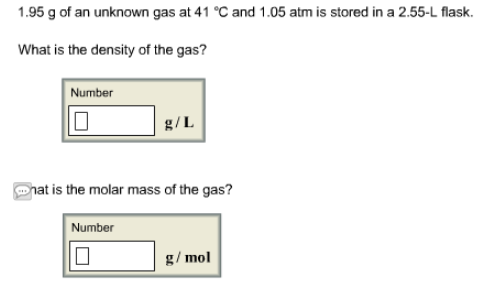# Problem: 1.95 g of an unknown gas at 41°C and 1.05 atm is stored in a 2.55-L flask. What is the density of the gas? What is the molar mass of the gas?

###### FREE Expert Solution
93% (228 ratings)###### Problem Details

1.95 g of an unknown gas at 41°C and 1.05 atm is stored in a 2.55-L flask.

What is the density of the gas?

What is the molar mass of the gas?Frequently Asked Questions

What scientific concept do you need to know in order to solve this problem?

Our tutors have indicated that to solve this problem you will need to apply the The Ideal Gas Law: Density concept. You can view video lessons to learn The Ideal Gas Law: Density. Or if you need more The Ideal Gas Law: Density practice, you can also practice The Ideal Gas Law: Density practice problems.